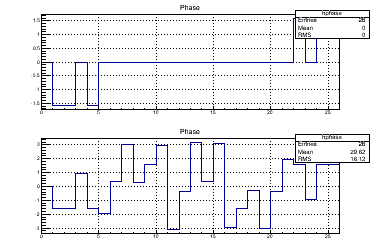# Phase in FFT

The code below is prepared using the tutorial FFT.C.
I fill the input data array input_double using the function fsin. I noticed that when I use a Float_t number instead of Double_t in fein->Eval(x) the phase plot has completely different appearance. Is that expected?
My root section and the code is below. To change type I remove comment from one line and add to another.
I’m using ROOT v5.34/18 on Mac OS X 10.9.4 “Mavericks”.

Thanks,
Andriy

fft\$ root

• ``````                                    *
``````
• ``````   W E L C O M E  to  R O O T       *
``````
• ``````                                    *
``````
• Version 5.34/18 14 March 2014 *
• ``````                                    *
``````
• ``````     [root.cern.ch](http://root.cern.ch)            *
``````
• ``````                                    *
``````

ROOT 5.34/18 (v5-34-18@v5-34-18, Mar 14 2014, 16:29:50 on macosx64)

CINT/ROOT C/C++ Interpreter version 5.18.00, July 2, 2010
Type ? for help. Commands must be C++ statements.
Enclose multiple statements between { }.
– Local rootlogon
// can = new TCanvas;
// can->Divide(1,2)
// can->cd(1)
)0x7fc57dc9a950
// .x fftphase.C+
Info in TMacOSXSystem::ACLiC: creating shared library /srv/zatserkl/work/fast/fft/./fftphase_C.so
// can->cd(2)
// .x fftphase.C+
Info in : it will be regenerated and reloaded!
Info in TMacOSXSystem::ACLiC: creating shared library /srv/zatserkl/work/fast/fft/./fftphase_C.so
Warning in TROOT::Append: Replacing existing TH1: hphase (Potential memory leak).
// can->cd()
// can->SaveAs(“fftphase.png”)
Info in TCanvas::Print: png file fftphase.png has been created

## fftphase.C

#include “TVirtualFFT.h”
#include “TF1.h”
#include “TH1.h”
#include “TMath.h”
#include “TCanvas.h”

void fftphase()
{
TF1 fsin = new TF1(“fsin”, "sin(x)+sin(2x)+sin(0.5x)+1", 0, 4TMath::Pi());
fsin->Draw();

Int_t N=26;
Double_t input_double;

for (Int_t i=0; i<N; i++){
// Double_t x = 4.*TMath::Pi()*i/N;
Float_t x = 4.*TMath::Pi()i/N;
input_double[i] = fsin->Eval(x);
}
TVirtualFFT
fftK = TVirtualFFT::FFT(1, &N, “R2C ES K”);
assert(fftK);
fftK->SetPoints(input_double);
fftK->Transform();

TH1F* hphase = new TH1F(“hphase”, “Phase”, N, 0, N);
TH1::TransformHisto(fftK, hphase, “PH”);
hphase->Draw();
}